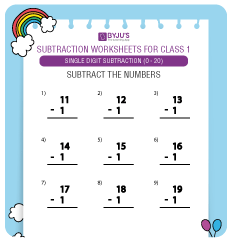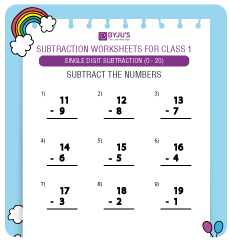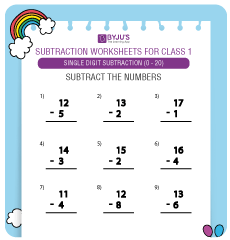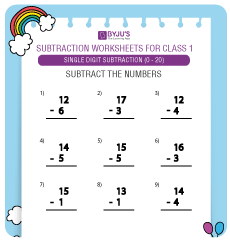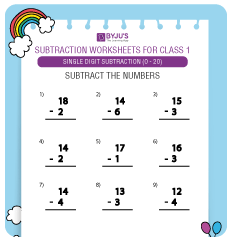# Single Digit Subtraction Worksheets for Grade 1 KidsHere, we bring to you Single Digit Subtraction Worksheets for Grade 1 kids. For a better practice of the concept, we are providing single digit subtraction worksheets for Grade 1 students. 1st Grade subtraction worksheets will help kids in gaining a sound command over the arithmetic operation of subtraction.

Subtraction is one of the most important arithmetic operations which are taught to kids in primary classes. Single-digit subtraction implies subtraction of one single-digit number from another single-digit number.

## Single Digit Subtraction Worksheets for Class 1(0 to 10):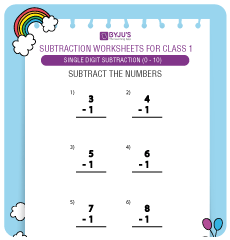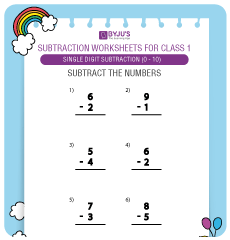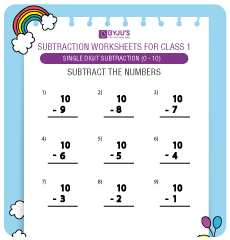## Single Digit Subtraction Worksheets for Class 1 (0 to 20):